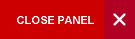# Department of Mathematics## Mathematics & Modelling Processes

This programme is designed to provide a strong background in analysis and to develop high technical abilities in the applications of mathematics to real world problems.. This programme will equip students for careers in teaching, industry, financial services, information technology, management and consultancy, government, and industrial research and development. A total of 60 advanced credits is required.

Compulsory Level 1 Mathematics & FD courses plus:
MATH1154 Intro. to Mathematical Software I (NEW)
STAT1001 Statistics for Scientists
MATH2401 Elements of Mathematical Analysis
MATH2410 A first course in Linear Algebra
MATH2421 Fourier Series & Integral Transforms
MATH2404 Introduction to Probability Theory
MATH2430 Linear Optimization
MATH2411 Introduction to Abstract Algebra
MATH2403 Multivariable Calculus
MATH2407 Stochastic Modelling
MATH2420 Ordinary Differential Equations
STAT2001 Inferential Statistics
MATH3155 Complex Variables
MATH3421 Partial Differential Equations
MATH3422 Mathematical Modelling
MATH3402 A course on Metric Space & Topology
MATH3424 Numerical Methods
MATH3423 Research Project
MATH3426 Numerical Solution of Ordinary Differential Equations (NEW)

PLUS 6 advanced credits from anywhere in the University

Electives - 9 credits from below:
MATH3401 - Intro. to the Theory of Integration